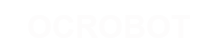#### 站点工具

learing:examples:virtualcolormixer

# Virtual Color Mixer

This example demonstrates how to send multiple values from the Arduino board to the computer. The readings from three potentiometers are used to set the red, green, and blue components of the background color of a Processing sketch or Max/MSP patch.

## Hardware Required

Arduino or Genuino Board 3 Analog Sensors (potentiometer, photocell, FSR, etc.) 3 10K ohm resistors hook-up wires breadboard

### Software Required

Processing or Max/MSP version 5 Circuit

This circuit uses three voltage divider sub-circuits to generate analog voltages from the force-sensing resistors. a voltage divider has two resistors in series, dividing the voltage proportionally to their values.

Click on the image to enlarge

image developed using Fritzing. For more circuit examples, see the Fritzing project page

Schematic

Click on the image to enlarge

#### Code

The sensor values are sent from the Arduino to the computer as ASCII-encoded decimal numbers. This means that each number is sent using the ASCII characters “0” through “9”. For the value “234” for example, three bytes are sent: ASCII “2” (binary value 50), ASCII “3” (binary value 51), and ASCII “4” (binary value 52).

```/*
This example reads three analog sensors (potentiometers are easiest)
and sends their values serially. The Processing and Max/MSP programs at the bottom
take those three values and use them to change the background color of the screen.

The circuit:

http://www.arduino.cc/en/Tutorial/VirtualColorMixer

created 2 Dec 2006
by David A. Mellis
modified 30 Aug 2011
by Tom Igoe and Scott Fitzgerald

This example code is in the public domain.
*/

const int redPin = A0;      // sensor to control red color
const int greenPin = A1;    // sensor to control green color
const int bluePin = A2;     // sensor to control blue color

void setup() {
Serial.begin(9600);
}

void loop() {
Serial.print(",");
Serial.print(",");
}```

Processing code for this example

```//  This example code is in the public domain.

import processing.serial.*;

float redValue = 0;        // red value
float greenValue = 0;      // green value
float blueValue = 0;       // blue value

Serial myPort;

void setup() {
size(200, 200);

// List all the available serial ports
// if using Processing 2.1 or later, use Serial.printArray()
println(Serial.list());

// I know that the first port in the serial list on my mac
// is always my  Arduino, so I open Serial.list().
// Open whatever port is the one you're using.
myPort = new Serial(this, Serial.list(), 9600);
// don't generate a serialEvent() unless you get a newline character:
myPort.bufferUntil('\n');
}

void draw() {
// set the background color with the color values:
background(redValue, greenValue, blueValue);
}

void serialEvent(Serial myPort) {
// get the ASCII string:

if (inString != null) {
// trim off any whitespace:
inString = trim(inString);
// split the string on the commas and convert the
// resulting substrings into an integer array:
float[] colors = float(split(inString, ","));
// if the array has at least three elements, you know
// you got the whole thing.  Put the numbers in the
// color variables:
if (colors.length >=3) {
// map them to the range 0-255:
redValue = map(colors, 0, 1023, 0, 255);
greenValue = map(colors, 0, 1023, 0, 255);
blueValue = map(colors, 0, 1023, 0, 255);
}
}
}```

Processing Code

Copy the Processing sketch from the code sample above. As you change the value of the analog sensors, the background color will change:

learing/examples/virtualcolormixer.txt · 最后更改: 2017/04/07 10:03 (外部编辑)

### 页面工具